# Menelaus theorem

(diff) ← Older revision | Latest revision (diff) | Newer revision → (diff)

A theorem on the relations between the lengths of the segments on the sides of a triangle determined by an intersecting straight line. It asserts that if the given line intersects the sides of a triangle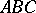(or their extensions) at the points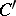,and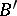, then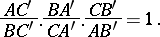Menelaus' theorem is a particular case of the Carnot theorem; it can be generalized to the case of a polygon. Thus, suppose that a straight lineintersects the edges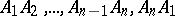of a polygon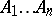at the respective points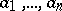. Then the following relation is valid: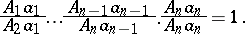The theorem was proved by Menelaus (1st century) and apparently it was known to Euclid (3rd century B.C.).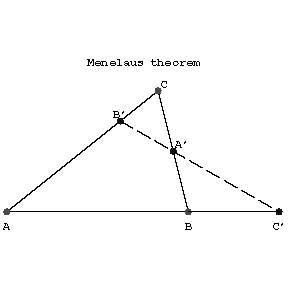Figure: m063400a